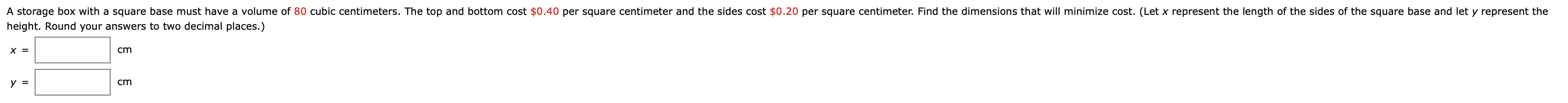# A storage box with a square base must have a volume of 80 cubic centimeters. The top and bottom cost $0.40 per square centimeter and the sides cost$0.20 per square centimeter. Find the dimensions that will minimize cost. (Let x represent the length of the sides of the square base and let y represent the height. Round your answers to two decimal places.) cm

Question

How do you answer the question in the attached image?help_outlineImage TranscriptioncloseA storage box with a square base must have a volume of 80 cubic centimeters. The top and bottom cost $0.40 per square centimeter and the sides cost$0.20 per square centimeter. Find the dimensions that will minimize cost. (Let x represent the length of the sides of the square base and let y represent the height. Round your answers to two decimal places.) cm fullscreen